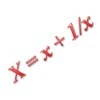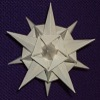Search by Topic

Resources tagged with Polynomial functions and their roots similar to System Speak:

Filter by: Content type:
Age range:
Challenge level:

There are 18 results

Broad Topics > Functions and Graphs > Polynomial functions and their rootsPolynomial Interpolation

Age 16 to 18 Challenge Level:

Can you fit polynomials through these points?Poly Fibs

Age 16 to 18 Challenge Level:

A sequence of polynomials starts 0, 1 and each poly is given by combining the two polys in the sequence just before it. Investigate and prove results about the roots of the polys.More Polynomial Equations

Age 16 to 18 Challenge Level:

Find relationships between the polynomials a, b and c which are polynomials in n giving the sums of the first n natural numbers, squares and cubes respectively.Common Divisor

Age 14 to 16 Challenge Level:

Find the largest integer which divides every member of the following sequence: 1^5-1, 2^5-2, 3^5-3, ... n^5-n.Telescoping Functions

Age 16 to 18

Take a complicated fraction with the product of five quartics top and bottom and reduce this to a whole number. This is a numerical example involving some clever algebra.Interpolating Polynomials

Age 16 to 18 Challenge Level:

Given a set of points (x,y) with distinct x values, find a polynomial that goes through all of them, then prove some results about the existence and uniqueness of these polynomials.Two Cubes

Age 14 to 16 Challenge Level:

Two cubes, each with integral side lengths, have a combined volume equal to the total of the lengths of their edges. How big are the cubes? [If you find a result by 'trial and error' you'll need to. . . .Mechanical Integration

Age 16 to 18 Challenge Level:

To find the integral of a polynomial, evaluate it at some special points and add multiples of these values.Polynomial Relations

Age 16 to 18 Challenge Level:

Given any two polynomials in a single variable it is always possible to eliminate the variable and obtain a formula showing the relationship between the two polynomials. Try this one.Janine's Conjecture

Age 14 to 16 Challenge Level:

Janine noticed, while studying some cube numbers, that if you take three consecutive whole numbers and multiply them together and then add the middle number of the three, you get the middle number. . . .Root to Poly

Age 14 to 16 Challenge Level:

Find the polynomial p(x) with integer coefficients such that one solution of the equation p(x)=0 is $1+\sqrt 2+\sqrt 3$.The Why and How of Substitution

Age 16 to 18

Step back and reflect! This article reviews techniques such as substitution and change of coordinates which enable us to exploit underlying structures to crack problems.An Introduction to Galois Theory

Age 16 to 18

This article only skims the surface of Galois theory and should probably be accessible to a 17 or 18 year old school student with a strong interest in mathematics.Fibonacci Fashion

Age 16 to 18 Challenge Level:

What have Fibonacci numbers to do with solutions of the quadratic equation x^2 - x - 1 = 0 ?Agile Algebra

Age 16 to 18 Challenge Level:

Observe symmetries and engage the power of substitution to solve complicated equations.Symmetrically So

Age 16 to 18 Challenge Level:

Exploit the symmetry and turn this quartic into a quadratic.